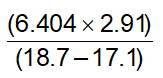# Problem: Perform the following mathematical operations and express the result to the correct number of significant figures.

###### Problem Details

Perform the following mathematical operations and express the result to the correct number of significant figures.Frequently Asked Questions

What scientific concept do you need to know in order to solve this problem?

Our tutors have indicated that to solve this problem you will need to apply the Multiplication and Division Operations concept. You can view video lessons to learn Multiplication and Division Operations. Or if you need more Multiplication and Division Operations practice, you can also practice Multiplication and Division Operations practice problems.

What is the difficulty of this problem?

Our tutors rated the difficulty ofPerform the following mathematical operations and express th...as low difficulty.

How long does this problem take to solve?

Our expert Analytical Chemistry tutor, Jules took 1 minute and 30 seconds to solve this problem. You can follow their steps in the video explanation above.